﻿ 一种新的基于数据驱动的神经动态规划方法 A New Data-Driven Neural Dynamic Programming Algorithm

Artificial Intelligence and Robotics Research
Vol. 08  No. 02 ( 2019 ), Article ID: 29320 , 11 pages
10.12677/AIRR.2019.82006

A New Data-Driven Neural Dynamic Programming Algorithm

Xingke Li, Xuesong Chen

School of Applied Mathematics, Guangdong University of Technology, Guangzhou Guangdong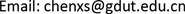Received: Feb. 27th, 2019; accepted: Mar. 13th, 2019; published: Mar. 20th, 2019ABSTRACT

A new data-driven neural dynamic programming method for model-free discrete-time nonlinear dynamic system is proposed in this paper. The residual of the Q-function and the control strategy are operated to be zero with the basis function through the inner product. Then the coefficients of the neural network are updated by the offline trained data and the online data. Finally the optimal control strategy is obtained and the convergence of this algorithm is proved.

Keywords:Optimal Control, Neural Dynamic Programming, Q-Function, Neural Network1. 引言

Bellman在1957年首次提出了动态规划方法。动态规划对于求解最优控制问题是一个行之有效的方法。该方法适应广泛：离散系统，连续系统，非线性系统，以及随机系统等等。但是该方法是一种逆向计算方法，随着解的时间维度增加，且由于控制变量维数大容易造成“维数灾难”问题。于是近年来，针对未知的复杂非线性系统的最优控制问题，自适应动态规划应运而生。与传统的动态规划相比，由于其采用函数近似结构来逼近系统模型，评价指标  ，和控制策略，避免了因时间逆向计算造成的“维数灾难”问题。自适应动态规划可用于大规模非线性系统  ，其应用也相当广泛；例如：机器人运动规划  ，无人驾驶汽车  ，污水处理系统  等等。

LIU等采用值迭代的自适应动态规划，并证明其收敛条件是迭代性能指标函数初始化为任意半正定函数  。根据此收敛条件，提出了一种基于自适应动态规划的协同优化算法  。该协同优化算法令迭代的残差快速减小，大幅提高了自适应动态规划的收敛速度。LIU等对于离散的非线性系统采用基于策略迭代的自适应动态规划方法  ，证明了该迭代控制律可以使系统稳定，并说明其是收敛的。LUO等则分别对于连续时间非线性系统采用基于策略迭代的自适应动态规划方法  ，该策略迭代的实现使用基于神经网络的最小二乘方法并证明该方法是收敛的。文献  针对离散时间非线性系统，提出了策略梯度自适应动态规划算法，并证明了该算法中Q函数能收敛到最优值。

2. 问题描述

${x}_{k+1}=f\left({x}_{k}+{u}_{k}\right),\text{ }\text{ }k=0,1,2,\cdots$ (1)

${V}_{u}\left({x}_{0}\right)={\sum }_{l=0}^{\infty }P\left({x}_{l},{u}_{l}\right).$ (2)

3. 策略梯度自适应动态规划

${V}_{u}\left({x}_{k}\right)={\sum }_{l=k}^{\infty }P\left({x}_{l},{u}_{l}\right).$ (3)

${V}_{u}\left({x}_{k}\right)=P\left({x}_{k},{u}_{k}\right)+{V}_{u}\left({x}_{k\text{+1}}\right).$ (4)

${V}^{*}\left(x\right)=\underset{u}{\mathrm{min}}{V}_{u}\left(x\right).$ (5)

${u}^{\text{*}}\left(x\right)=\mathrm{arg}\underset{u}{\mathrm{min}}{V}_{u}\left({x}_{k}\right).$ (6)

${Q}_{u}\left({x}_{k},a\right)=P\left({x}_{k},a\right)+{\sum }_{l=k+\text{1}}^{\infty }P\left({x}_{l},{u}_{l}\right).$ (7)

${Q}_{u}\left({x}_{k},a\right)=P\left({x}_{k},a\right)+{Q}_{u}\left({x}_{k+1},u\right)=\text{}P\left({x}_{k},a\right)+{V}_{u}\left({x}_{k+1}\right).$ (8)

${Q}^{*}\left({x}_{k},a\right)={Q}_{{u}^{*}}\left({x}_{k},a\right)=P\left({x}_{k},a\right)+{V}^{*}\left({x}_{k+1}\right).$ (9)

${u}^{*}\left(x\right)=\text{}\mathrm{arg}\underset{u}{\mathrm{min}}{V}_{u}\left(x\right)=\mathrm{arg}\underset{a}{\mathrm{min}}{Q}^{*}\left(x,a\right).$ (10)

${Q}^{\left(i\right)}\left({x}_{k},a\right)=P\left({x}_{k},a\right)+{Q}^{\left(i\right)}\left({x}_{k\text{+1}},{u}^{\left(i\right)}\right).$ (11)

${u}^{\left(i+1\right)}\left(x\right)={u}^{\left(i\right)}\left(x\right)-{\alpha {\nabla }_{a}{Q}^{\left(i\right)}\left(x,a\right)|}_{a={u}^{\left(i\right)}}.$ (12)

$\begin{array}{c}H\left({x}_{k},u,V\right)=V\left({x}_{k+1}\right)-V\left({x}_{k}\right)+P\left({x}_{k},u\right)\\ =V\left(f\left({x}_{k},u\right)\right)-V\left({x}_{k}\right)+P\left({x}_{k},u\right).\end{array}$ (13)

${V}^{\left(i\right)}\left({x}_{k}\right)={V}_{{u}^{\left(i\right)}}\left({x}_{k}\right)={\sum }_{l=k}^{\infty }P\left({x}_{l},{u}^{\left(i\right)}\left({x}_{l}\right)\right)$

1)2) ${u}^{\left(i\right)}\in U\left(X\right)$

$\begin{array}{l}H\left({x}_{k},u,{V}^{\left(i\right)}\right)=H\left({x}_{k},{u}^{\left(i\right)},{V}^{\left(i\right)}\right)+{\left(u-{u}^{\left(i\right)}\right)}^{\text{T}}{\nabla }_{u}H\left({x}_{k},{u}^{\left(i\right)},{V}^{\left(i\right)}\right)\\ \text{ }\text{ }\text{ }\text{ }\text{ }\text{ }\text{ }\text{ }\text{ }\text{ }\text{ }\text{ }\text{ }\text{ }\text{ }\text{ }\text{ }\text{ }\text{ }\text{ }\text{ }\text{ }\text{ }\text{ }\text{ }\text{ }\text{ }\text{ }\text{ }\text{ }\text{ }\text{ }\text{ }\text{ }\text{ }\text{ }\text{ }\text{ }\text{ }\text{ }\text{ }\text{ }\text{ }\text{ }\text{ }\text{ }\text{ }\text{ }\text{ }\text{ }\text{ }\text{ }\text{ }\text{ }\text{ }\text{ }\text{ }\text{ }\text{ }\text{ }\text{ }\text{ }\text{ }\text{ }\text{ }+\frac{1}{2}{\left(u-{u}^{\left(i\right)}\right)}^{\text{T}}{\nabla }_{uu}H\left({x}_{k},{u}^{\left(i\right)},{V}^{\left(i\right)}\right)\left(u-{u}^{\left(i\right)}\right)+h\left(u\right).\end{array}$ (14)

${\nabla }_{a}{Q}^{\left(i\right)}\left({x}_{k},a\right)={\nabla }_{a}{V}^{\left(i\right)}\left(f\left({x}_{k},a\right)\right)+{\nabla }_{a}W\left(a\right)={\nabla }_{u}H\left({x}_{k},u,{V}^{\left(i\right)}\right).$ (15)

${\nabla }_{aa}{Q}^{\left(i\right)}\left({x}_{k},a\right)={\nabla }_{aa}{V}^{\left(i\right)}\left(f\left({x}_{k},a\right)\right)+{\nabla }_{aa}W\left(a\right)={\nabla }_{uu}H\left({x}_{k},u,{V}^{\left(i\right)}\right).$ (16)

$\begin{array}{l}H\left({x}_{k},{u}^{\left(i+1\right)},{V}^{\left(i\right)}\right)\\ =H\left({x}_{k},{u}^{\left(i\right)},{V}^{\left(i\right)}\right)+{\left({u}^{\left(i+1\right)}-{u}^{\left(i\right)}\right)}^{\text{T}}{\nabla }_{u}H\left({x}_{k},{u}^{\left(i\right)},{V}^{\left(i\right)}\right)\\ \text{\hspace{0.17em}}\text{\hspace{0.17em}}+\frac{1}{2}{\left({u}^{\left(i+1\right)}-{u}^{\left(i\right)}\right)}^{\text{T}}{\nabla }_{uu}H\left({x}_{k},{u}^{\left(i\right)},{V}^{\left(i\right)}\right)\left({u}^{\left(i+1\right)}-{u}^{\left(i\right)}\right)+h\left({u}^{\left(i+1\right)}\right)\end{array}$

$\begin{array}{l}=H\left({x}_{k},{u}^{\left(i\right)},{V}^{\left(i\right)}\right)-\alpha {\nabla }_{a}^{T}{Q}^{\left(i\right)}\left({x}_{k},{u}^{\left(i\right)}\right){\nabla }_{u}H\left({x}_{k},{u}^{\left(i\right)},{V}^{\left(i\right)}\right)\\ \text{\hspace{0.17em}}\text{\hspace{0.17em}}+\frac{1}{2}{\alpha }^{2}{\nabla }_{a}^{\text{T}}{Q}^{\left(i\right)}\left({x}_{k},{u}^{\left(i\right)}\right){\nabla }_{uu}H\left({x}_{k},{u}^{\left(i\right)},{V}^{\left(i\right)}\right){\nabla }_{a}{Q}^{\left(i\right)}\left({x}_{k},{u}^{\left(i\right)}\right)+h\left({u}^{\left(i+1\right)}\right)\\ =H\left({x}_{k},{u}^{\left(i\right)},{V}^{\left(i\right)}\right)-\alpha {\nabla }_{a}^{\text{T}}H\left({x}_{k},{u}^{\left(i\right)},{V}^{\left(i\right)}\right){\nabla }_{u}H\left({x}_{k},{u}^{\left(i\right)},{V}^{\left(i\right)}\right)\\ \text{\hspace{0.17em}}\text{\hspace{0.17em}}+\frac{1}{2}{\alpha }^{2}{\nabla }_{a}^{T}H\left({x}_{k},{u}^{\left(i\right)},{V}^{\left(i\right)}\right){\nabla }_{uu}H\left({x}_{k},{u}^{\left(i\right)},{V}^{\left(i\right)}\right)H\left({x}_{k},{u}^{\left(i\right)},{V}^{\left(i\right)}\right)+h\left({u}^{\left(i+1\right)}\right).\end{array}$

$A={\nabla }_{u}^{\text{T}}H{\nabla }_{uu}H{\nabla }_{u}H,B={\nabla }_{u}^{\text{T}}H{\nabla }_{u}H,C=h$ ，且 $A\ge 0$ ，则：

$H\left({x}_{k},{u}^{\left(i+1\right)},{V}^{\left(i\right)}\right)=H\left({x}_{k},{u}^{\left(i\right)},{V}^{\left(i\right)}\right)+\frac{1}{2}A{\alpha }^{2}-B\alpha +C.$ (17)

$\frac{\text{1}}{\text{2}}A{\alpha }^{2}-B\alpha +C\le 0.$ (18)

$H\left({x}_{k},{u}^{\left(i+1\right)},{V}^{\left(i\right)}\right)\le H\left({x}_{k},{u}^{\left(i\right)},{V}^{\left(i\right)}\right)$ 。根据Hamiltonian函数定义知： $H\left({x}_{k},{u}^{\left(i\right)},{V}^{\left(i\right)}\right)=0$

2) 假设 ${u}^{\left(i\right)}\left(x\right)\in U\left(X\right)$ ，对于系统 ${x}_{k+1}=f\left({x}_{k},{u}^{\left(i+1\right)}\right)$ ，则对于Lyapunov函数 ${V}^{\left(i\right)}\left({x}_{k}\right)$ 有：

$\begin{array}{c}\Delta {V}^{\left(i\right)}\left({x}_{k}\right)={V}^{\left(i\right)}\left(f\left({x}_{k},{u}^{\left(i+1\right)}\right)\right)-{V}^{\left(i\right)}\left({x}_{k}\right)\\ ={V}^{\left(i\right)}\left(f\left({x}_{k},{u}^{\left(i+1\right)}\right)\right)-{V}^{\left(i\right)}\left({x}_{k}\right)+P\left({x}_{k},{u}^{\left(i+1\right)}\right)-P\left({x}_{k},{u}^{\left(i+1\right)}\right)\\ =H\left({x}_{k},{u}^{\left(i+1\right)},{V}^{\left(i\right)}\right)-P\left({x}_{k},{u}^{\left(i+1\right)}\right).\end{array}$ (19)

$H\left({x}_{k},{u}^{\left(i+1\right)},{V}^{\left(i\right)}\right)\le 0$ ，知： $\Delta {V}^{\left(i\right)}\left({x}_{k}\right)\le -P\left({x}_{k},{u}^{\left(i+1\right)}\right)\le 0$ 。即： ${V}^{\left(i\right)}\left(f\left({x}_{k},{u}^{\left(i+1\right)}\right)\right)\le {V}^{\left(i\right)}\left({x}_{k}\right)<\infty$

2) $\underset{i\to \infty }{\mathrm{lim}}{Q}^{\left(i\right)}\left(x,a\right)={Q}^{*}\left(x,a\right)$

$\begin{array}{c}{Q}^{\left(i\right)}\left({x}_{k},{u}^{\left(i+1\right)}\right)=P\left({x}_{k},{u}^{\left(i+1\right)}\right)+{V}^{\left(i\right)}\left(f\left({x}_{k},{u}^{\left(i+1\right)}\right)\right)\\ =P\left({x}_{k},{u}^{\left(i+1\right)}\right)+{V}^{\left(i\right)}\left(f\left({x}_{k},{u}^{\left(i+1\right)}\right)\right)-{V}^{\left(i\right)}\left({x}_{k}\right)+{V}^{\left(i\right)}\left({x}_{k}\right)\\ =H\left({x}_{k},{u}^{\left(i+1\right)},{V}^{\left(i\right)}\right)+{V}^{\left(i\right)}\left({x}_{k}\right)\\ \le H\left({x}_{k},{u}^{\left(i\right)},{V}^{\left(i\right)}\right)+{V}^{\left(i\right)}\left({x}_{k}\right)\\ ={V}^{\left(i\right)}\left({x}_{k}\right).\end{array}$ (20)

$\begin{array}{c}{V}^{\left(i+1\right)}\left({x}_{k}\right)=P\left({x}_{k},{u}^{\left(i+1\right)}\right)+{V}^{\left(i\right)}\left({x}_{k+1}\right)-{V}^{\left(i\right)}\left({x}_{k+1}\right)+{V}^{\left(i+1\right)}\left({x}_{k+1}\right)\\ ={Q}^{\left(i\right)}\left({x}_{k},{u}^{\left(i+1\right)}\right)-{V}^{\left(i\right)}\left({x}_{k+1}\right)+{V}^{\left(i+1\right)}\left({x}_{k+1}\right)\\ \le {V}^{\left(i\right)}\left({x}_{k}\right)-{V}^{\left(i\right)}\left({x}_{k+1}\right)+{V}^{\left(i+1\right)}\left({x}_{k+1}\right)\\ =P\left({x}_{k},{u}^{\left(i\right)}\right)+{V}^{\left(i+1\right)}\left({x}_{k+1}\right)\\ \le P\left({x}_{k},{u}^{\left(i\right)}\right)+P\left({x}_{k+1},{u}^{\left(i\right)}\right)+{V}^{\left(i+1\right)}\left({x}_{k+2}\right)\\ \le {\sum }_{l=k}^{\infty }P\left({x}_{l},{u}^{\left(i\right)}\right)={V}^{\left(i\right)}\left({x}_{k}\right).\end{array}$ (21)

$\begin{array}{c}{Q}^{\left(i+1\right)}\left({x}_{k},a\right)=P\left({x}_{k},a\right)+{V}^{\left(i+1\right)}\left({x}_{k+1}\right)\\ \le P\left({x}_{k},a\right)+{V}^{\left(i\right)}\left({x}_{k+1}\right)\\ ={Q}^{\left(i\right)}\left({x}_{k},a\right).\end{array}$ (22)

$\begin{array}{c}{Q}^{\left(i\right)}\left({x}_{k},a\right)=P\left({x}_{k},a\right)+{V}^{\left(i\right)}\left({x}_{k+1}\right)\\ \ge P\left({x}_{k},a\right)+{V}^{*}\left({x}_{k+1}\right)\\ ={Q}^{\text{*}}\left({x}_{k},a\right).\end{array}$ (23)

2) 由式(12)知，当i趋于 $\infty$ 时有：

${u}^{\infty }={u}^{\infty }-{\alpha {\nabla }_{a}{Q}^{\infty }\left(x,a\right)|}_{a={u}^{\infty }}.$ (24)

${\alpha {\nabla }_{a}{Q}^{\infty }\left(x,a\right)|}_{a={u}^{\infty }}=0.$ (25)

 (26)

$\text{ }{{\nabla }_{a}W\left(a\right)|}_{a={u}^{\infty }}+{{\nabla }_{a}{V}^{\infty }\left(f\left({x}_{k},a\right)\right)|}_{a={u}^{\infty }}=0.$ (27)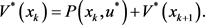(28)

${{\nabla }_{u}W\left(u\right)|}_{u={u}^{*}}+{{\nabla }_{u}{V}^{\text{*}}\left(f\left({x}_{k},u\right)\right)|}_{u={u}^{*}}=0.$ (29)

${V}^{\infty }\left(x\right)={V}^{\text{*}}\left(x\right).$ (30)

$\begin{array}{c}{Q}^{\infty }\left({x}_{k},a\right)=P\left({x}_{k},a\right)+{V}^{\infty }\left({x}_{k+1}\right)\\ =P\left({x}_{k},a\right)+{V}^{*}\left({x}_{k+1}\right)\\ ={Q}^{*}\left({x}_{k},a\right).\end{array}$ (31)

$\underset{i\to \infty }{\mathrm{lim}}{Q}^{\left(i\right)}\left(x,a\right)={Q}^{*}\left(x,a\right)$

4. 基于数据驱动的神经动态规划及其实现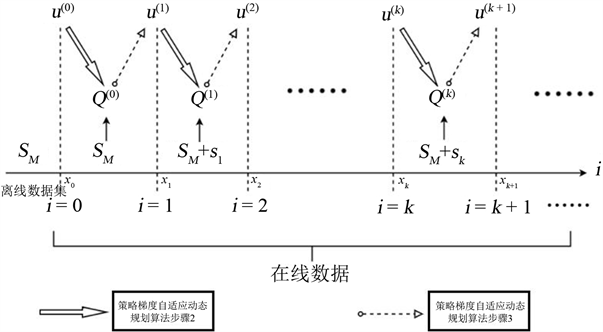Figure 2. Collect offline data sets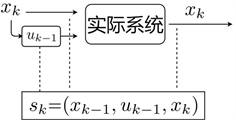Figure 3. Collect online data

4.1. 神经动态规划的实现设计

$u\left(x\right)={\sum }_{j=1}^{\infty }{\upsilon }_{j}{\varphi }_{j}\left(x\right)\text{ }\text{ }.$ (32)

$Q\left(x,a\right)={\sum }_{j=1}^{\infty }{\theta }_{j}{\psi }_{j}\left(x,a\right)\text{ }\text{ }.$ (33)

 (34)

${\stackrel{¯}{Q}}^{\left(i\right)}\left(x,a\right)={\sum }_{j=1}^{{L}_{2}}{\theta }_{j}^{\left(i\right)}{\psi }_{j}\left(x,a\right)={\Psi }_{L}^{\text{T}}\left(x,a\right){\theta }^{\left(i\right)}.$ (35)

${\stackrel{^}{u}}^{\left(i\right)}\left(x\right)={\sum }_{j=1}^{{L}_{1}}{\stackrel{^}{\upsilon }}_{j}^{\left(i\right)}{\varphi }_{j}\left(x\right)={\Phi }_{L}^{\text{T}}\left(x\right){\stackrel{^}{\upsilon }}^{\left(i\right)}.$ (36)

${\stackrel{^}{Q}}^{\left(i\right)}\left(x,a\right)={\sum }_{j=1}^{{L}_{2}}{\stackrel{^}{\theta }}_{j}^{\left(i\right)}{\psi }_{j}\left(x,a\right)={\Psi }_{L}^{\text{T}}\left(x,a\right){\stackrel{^}{\theta }}^{\left(i\right)}.$ (37)

$\begin{array}{c}{\sigma }_{Q}^{\left(i\right)}\left(x,a,\stackrel{˜}{x}\right)={\stackrel{^}{Q}}^{\left(i\right)}\left(x,a\right)-{\stackrel{^}{Q}}^{\left(i\right)}\left(\stackrel{˜}{x},{\stackrel{^}{u}}^{\left(i\right)}\right)-P\left(x,a\right)\\ ={\Psi }_{L}^{\text{T}}\left(x,a\right){\stackrel{^}{\theta }}^{\left(i\right)}-{\Psi }_{L}^{\text{T}}\left(\stackrel{˜}{x},{\stackrel{^}{u}}^{\left(i\right)}\right){\stackrel{^}{\theta }}^{\left(i\right)}-P\left(x,a\right)\\ ={\Psi }_{L}^{\text{T}}\left(x,a\right){\stackrel{^}{\theta }}^{\left(i\right)}-{\Psi }_{L}^{\text{T}}\left(\stackrel{˜}{x},{\Phi }_{L}^{\text{T}}\left(\stackrel{˜}{x}\right){\stackrel{^}{\upsilon }}^{\left(i\right)}\right){\stackrel{^}{\theta }}^{\left(i\right)}-P\left(x,a\right).\end{array}$ (38)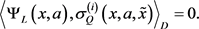(39)

$\begin{array}{l}\left[{〈{\Psi }_{L}\left(x,a\right),{\Psi }_{L}^{\text{T}}\left(x,a\right)〉}_{D}-{〈{\Psi }_{L}\left(x,a\right),{\Psi }_{L}^{\text{T}}\left(\stackrel{˜}{x},{\Phi }_{L}^{\text{T}}\left(\stackrel{˜}{x}\right){\stackrel{^}{\upsilon }}^{\left(i\right)}\right)〉}_{D}\right]{\stackrel{^}{\theta }}^{\left(i\right)}\\ -{〈{\Psi }_{L}\left(x,a\right),P\left(x,a\right)〉}_{D}=0.\end{array}$ (40)(41)

$\begin{array}{c}{〈{\Psi }_{L}\left(x,a\right),{\Psi }_{L}^{\text{T}}\left(x,a\right)〉}_{D}={\int }_{D}{\Psi }_{L}\left(x,a\right){\Psi }_{L}^{\text{T}}\left(x,a\right)\text{d}\left(x,a\right)\\ =\frac{{I}_{D}}{M}{\sum }_{l=1}^{M}{\Psi }_{L}\left({x}_{l},{a}_{l}\right){\Psi }_{L}^{\text{T}}\left({x}_{l},{a}_{l}\right)\\ =\frac{{I}_{D}}{M}{\eta }_{0}.\end{array}$ (42)

${〈{\Psi }_{L}\left(x,a\right),{\Psi }_{L}^{\text{T}}\left(\stackrel{˜}{x},{\Phi }_{L}^{\text{T}}\left(\stackrel{˜}{x}\right){\stackrel{^}{\upsilon }}^{\left(0\right)}\right)〉}_{D}=\frac{{I}_{D}}{M}{\rho }_{0}.$ (43)

 (44)

${\xi }_{0}={\sum }_{l=1}^{M}{\Psi }_{L}\left({x}_{l},{a}_{l}\right)P\left({x}_{l},{a}_{l}\right)$ 。则可得：

${\stackrel{^}{\theta }}^{\left(0\right)}={\left({\eta }_{0}-{\rho }_{0}\right)}^{-1}{\xi }_{0}.$ (45)

${〈{\Psi }_{L}\left(x,a\right),{\Psi }_{L}^{\text{T}}\left(x,a\right)〉}_{D}=\frac{{I}_{D}}{M+1}{\eta }_{k}.$ (46)

${〈{\Psi }_{L}\left(x,a\right),{\Psi }_{L}^{\text{T}}\left(\stackrel{˜}{x},{\Phi }_{L}^{\text{T}}\left(\stackrel{˜}{x}\right){\stackrel{^}{\upsilon }}^{\left(k\right)}\right)〉}_{D}=\frac{{I}_{D}}{M+1}{\rho }_{k}.$ (47)

${〈{\Psi }_{L}\left(x,a\right),P\left(x,a\right)〉}_{D}=\frac{{I}_{D}}{M\text{+1}}{\xi }_{k}.$ (48)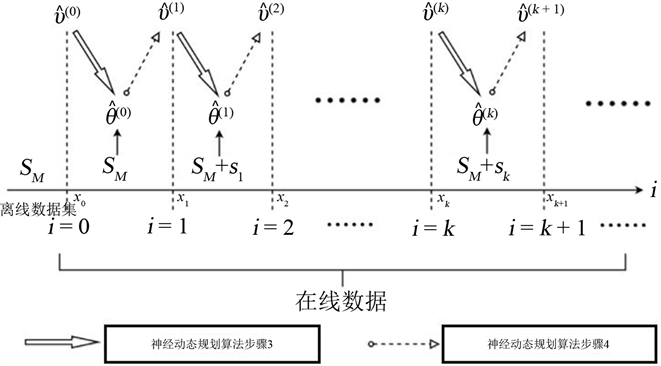Figure 4. Neural dynamic programming algorithm

${\rho }_{k}={\sum }_{l=1}^{M}{\Psi }_{L}\left({x}_{l},{a}_{l}\right){\Psi }_{L}^{\text{T}}\left({\stackrel{˜}{x}}_{l},{\Phi }_{L}^{\text{T}}\left({\stackrel{˜}{x}}_{l}\right){\stackrel{^}{\upsilon }}^{\left(k\right)}\right)+{\Psi }_{L}\left({x}_{k-1},{a}_{k-1}\right){\Psi }_{L}^{\text{T}}\left({x}_{k},{u}_{k}\right).$

${\stackrel{^}{\theta }}^{\left(k\right)}={\left({\eta }_{k}-{\rho }_{k}\right)}^{-1}{\xi }_{k}.$ (49)

$\begin{array}{c}{\sigma }_{u}^{\left(i\right)}\left(x\right)={u}^{\left(i+1\right)}\left(x\right)-{u}^{\left(i\right)}\left(x\right)+{\alpha {\nabla }_{a}{Q}^{\left(i\right)}\left(x,a\right)|}_{a={u}^{\left(i\right)}}\\ ={\Phi }_{L}^{\text{T}}\left(x\right){\stackrel{^}{\upsilon }}^{\left(i+1\right)}-{\Phi }_{L}^{\text{T}}\left(x\right){\stackrel{^}{\upsilon }}^{\left(i\right)}+\alpha {\nabla }_{a}{\Phi }_{L}^{\text{T}}\left(x,{\Phi }_{L}^{\text{T}}\left(x\right){\stackrel{^}{\upsilon }}^{\left(i\right)}\right){\stackrel{^}{\theta }}^{\left(i\right)}.\end{array}$ (50)

 (51)

$\begin{array}{l}{〈{\Phi }_{L}\left(x\right),{\Phi }_{L}^{\text{T}}\left(x\right)〉}_{X}{\stackrel{^}{\upsilon }}^{\left(i+1\right)}-{〈{\Phi }_{L}\left(x\right),{\Phi }_{L}^{\text{T}}\left(x\right)〉}_{X}{\stackrel{^}{\upsilon }}^{\left(i\right)}\\ \text{ }+\alpha {〈{\Phi }_{L}\left(x\right),{\nabla }_{a}{\Phi }_{L}^{\text{T}}\left(x,{\Phi }_{L}^{\text{T}}\left(x\right){\stackrel{^}{\upsilon }}^{\left(i\right)}\right)〉}_{X}{\stackrel{^}{\theta }}^{\left(i\right)}=0.\end{array}$ (52)

${\stackrel{^}{\upsilon }}^{\left(i+1\right)}={\stackrel{^}{\upsilon }}^{\left(i\right)}-\alpha {〈{\Phi }_{L}\left(x\right),{\Phi }_{L}^{\text{T}}\left(x\right)〉}_{X}^{-1}×{〈{\Phi }_{L}\left(x\right),{\nabla }_{a}{\Phi }_{L}^{\text{T}}\left(x,{\Phi }_{L}^{\text{T}}\left(x\right){\stackrel{^}{\upsilon }}^{\left(i\right)}\right)〉}_{X}{\stackrel{^}{\theta }}^{\left(i\right)}.$ (53)

 (54)

${〈{\Phi }_{L}\left(x\right),{\nabla }_{a}{\Psi }_{L}^{\text{T}}\left(x,{\Phi }_{L}^{\text{T}}\left(x\right){\stackrel{^}{\upsilon }}^{\left(k\right)}\right)〉}_{X}=\frac{{I}_{X}}{M+1}{F}_{k}.$ (55)

${F}_{k}={\sum }_{l=1}^{M}{\Phi }_{L}\left({x}_{l}\right){\nabla }_{a}{\Psi }_{L}^{\text{T}}\left({x}_{l},{\Phi }_{L}^{\text{T}}\left({x}_{l}\right){\stackrel{^}{\upsilon }}^{\left(k\right)}\right)+{\Phi }_{L}\left({x}_{k}\right){\nabla }_{a}{\Psi }_{L}^{\text{T}}\left({x}_{k},{\Phi }_{L}^{\text{T}}\left({x}_{k}\right){\stackrel{^}{\upsilon }}^{\left(k\right)}\right)$ 。则由(53)可得：

${\stackrel{^}{\upsilon }}^{\left(k+1\right)}={\stackrel{^}{\upsilon }}^{\left(k\right)}-\alpha {\Gamma }_{k}^{-1}{F}_{k}{\stackrel{^}{\theta }}^{\left(k\right)}.$ (56)

4.2. 神经动态规划算法

5. 结束语

A New Data-Driven Neural Dynamic Programming Algorithm[J]. 人工智能与机器人研究, 2019, 08(02): 46-56. https://doi.org/10.12677/AIRR.2019.82006

1. 1. 张化光, 张欣, 罗艳红, 杨珺. 自适应动态规划综述[J]. 自动化学报, 2013, 39(4): 303-311.

2. 2. 林小峰, 丁强. 基于评价网络近似误差的自适应动态规划优化控制[J]. 控制与决策, 2015, 30(3): 495-499.

3. 3. Lakovos, M., Simone, B., Elias, B.K. and Petros, A.L. (2017) Adaptive Optimal Control for Large-Scale Nonlinear Systems. IEEE Transactions on Automatica Control, 62, 5567-5577. https://doi.org/10.1109/TAC.2017.2684458

4. 4. 赵金刚, 戈新生. 基于动态规划的机器人运动规划最优控制[J]. 控制工程, 2017, 24(11): 2374-2379.

5. 5. 田涛涛, 侯忠生, 刘世达, 邓志东. 基于无模型自适应动态规划的无人驾驶汽车横向控制方法[J]. 自动化学报, 2017, 43(11): 1931-1940.

6. 6. 乔俊飞, 王亚清, 柴伟. 基于迭代ADP算法的污水处理过程最优控制[J]. 北京工业大学学报, 2018, 44(2): 200-206.

7. 7. 刘毅, 章云. 基于值迭代的自适应动态规划的收敛条件[J]. 广东工业大学学报, 2017, 34(5): 10-14.

8. 8. 刘毅, 章云. 一种基于自适应动态规划的协同优化算法[J]. 广东工业大学学报, 2017, 34(6): 15-19.

9. 9. Liu, D.R. and Wei Q.L. (2014) Policy Iteration Adaptive Dynamic Programming Algorithm for Dis-crete-Time Nonlinear Systems. IEEE Transactions on Neural Networks Learning Systems, 2014, 25, 621-634. https://doi.org/10.1109/TNNLS.2013.2281663

10. 10. Luo, B., Wu, H.N., Huang, T.W. and Liu, D.R. (2014) Data Based Approximate Policy Iteration for Affine Nonlinear Continuous-Time Optimal Control Design. Automatica, 50, 3281-3290. https://doi.org/10.1016/j.automatica.2014.10.056

11. 11. Luo, B., Liu, D.R., Wu, H.N., Wang, D. and Lewis, F.L. (2017) Policy Gradient Adaptive Dynamic Programming for Data-Based Optimal Control. IEEE Transactions on Cybernrtics, 47, 3341-3354. https://doi.org/10.1109/TCYB.2016.2623859

12. 12. 王鼎, 穆朝絮, 刘德荣. 基于迭代神经动态规划的数据驱动非线性近似最优调节[J]. 自动化学报, 2017, 43(3): 366-375.